# Purpose of this seminar

This seminar introduces how to use the R ggplot2 package, particularly for producing statistical graphics for data analysis.

• First the underlying grammar (system) of graphics is introduced with examples.
• Then, we’ll practice using the elements of the grammar by creating a customized graph.
• Finally, we’ll address common issues that arise when creating statistical graphics.

Text in this font signifies R code or variables in a data set

Text that appears like this represents an instruction to practice ggplot2 coding

# Seminar packages

Next we load the packages into the current R session with library(). In addition to ggplot2, we load package MASS (installed with R) for data sets.

#load libraries into R session
library(ggplot2)
library(MASS)

Please use library() to load packages ggplot2 and MASS.

# The ggplot2 package

• produces layered statistical graphics
• uses an underlying “grammar” to build graphs layer-by-layer rather than providing premade graphs
• is easy enough to use without any exposure to the underlying grammar, but is even easier to use once you know the grammar
• allows the user to build a graph from concepts rather than recall of commands and options

# ggplot2 documentation

https://ggplot2.tidyverse.org/reference/

The official reference webpage for ggplot2 has help files for its many functions an operators. Many examples are provided in each help file.

# What is a grammar of graphics?

A grammar of a language defines the rules of structuring words and phrases into meaningful expressions.

A grammar of graphics defines the rules of structuring mathematic and aesthetic elements into a meaningful graph.

Leland Wilkinson (2005) designed the grammar upon which ggplot2 is based.

# Elements of grammar of graphics

1. Data: variables mapped to aesthetic features of the graph.
2. Geoms: objects/shapes on the graph.
3. Stats: statistical transformations that summarize data,(e.g mean, confidence intervals).
4. Scales: mappings of aesthetic values to data values. Legends and axes visualize scales.
5. Coordinate systems: the plane on which data are mapped on the graphic.
6. Faceting: splitting the data into subsets to create multiple variations of the same graph (paneling).

# The Sitka dataset

To practice using the grammar of graphics, we will use the Sitka dataset (from the MASS package).

Note: Data sets that are loaded into R with a package are immediately available for use. To see the object appear in RStudio’s Environment pane (so you can click to view it), run data() on the data set, and then another function like str() on the data set.

Use data() and then str() on Sitka to make it appear in the Environment pane.

The Sitka dataset describes the growth of trees over time, some of which were grown in ozone-enriched chambers. The data frame contains 395 rows of the following 4 columns:

• size: numeric, log of size (height times diameter2)
• Time: numeric, time of measurement (days since January 1, 1988)
• tree: integer, tree id
• treat: factor, treatment group, 2 levels=“control” and “ozone”

Here are the first few rows of Sitka:

size Time tree treat
4.51 152 1 ozone
4.98 174 1 ozone
5.41 201 1 ozone
5.90 227 1 ozone
6.15 258 1 ozone
4.24 152 2 ozone

# The ggplot() function and aesthetics

All graphics begin with specifying the ggplot() function (Note: not ggplot2, the name of the package)

In the ggplot() function we specify the data set that holds the variables we will be mapping to aesthetics, the visual properties of the graph. The data set must be a data.frame object.

Example syntax for ggplot() specification (italicized words are to be filled in by you):

ggplot(data, aes(x=xvar, y=yvar))

• data: name of the data.frame that holds the variables to be plotted
• x and y: aesthetics that position objects on the graph
• xvar and yvar: names of variables in data mapped to x and y

Notice that the aesthetics are specified inside aes(), which is itself nested inside of ggplot().

The aesthetics specified inside of ggplot() are inherited by subsequent layers:

# scatter plot of volume vs sales
ggplot(txhousing, aes(x=volume, y=sales)) +
geom_point()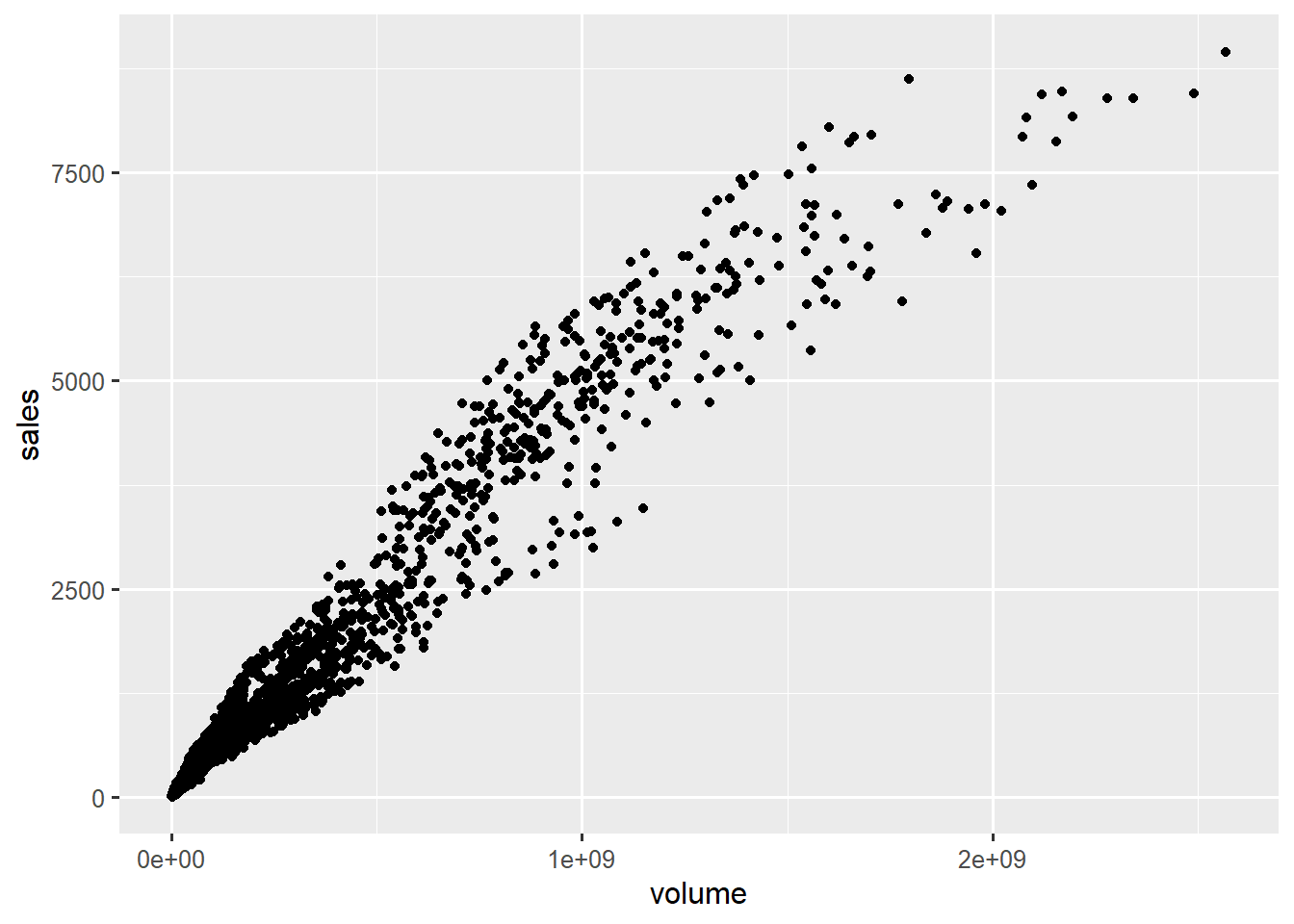geom_point() inherits x and y aesthetics

Initiate a graph of Time vs size by mapping Time to x and size to y from the data set Sitka.

Without any additional layers, no data will be plotted.

# Layers and overriding aesthetics

Specifying just x and y aesethetics alone will produce a plot with just the 2 axes.

ggplot(data = txhousing, aes(x=volume, y=sales))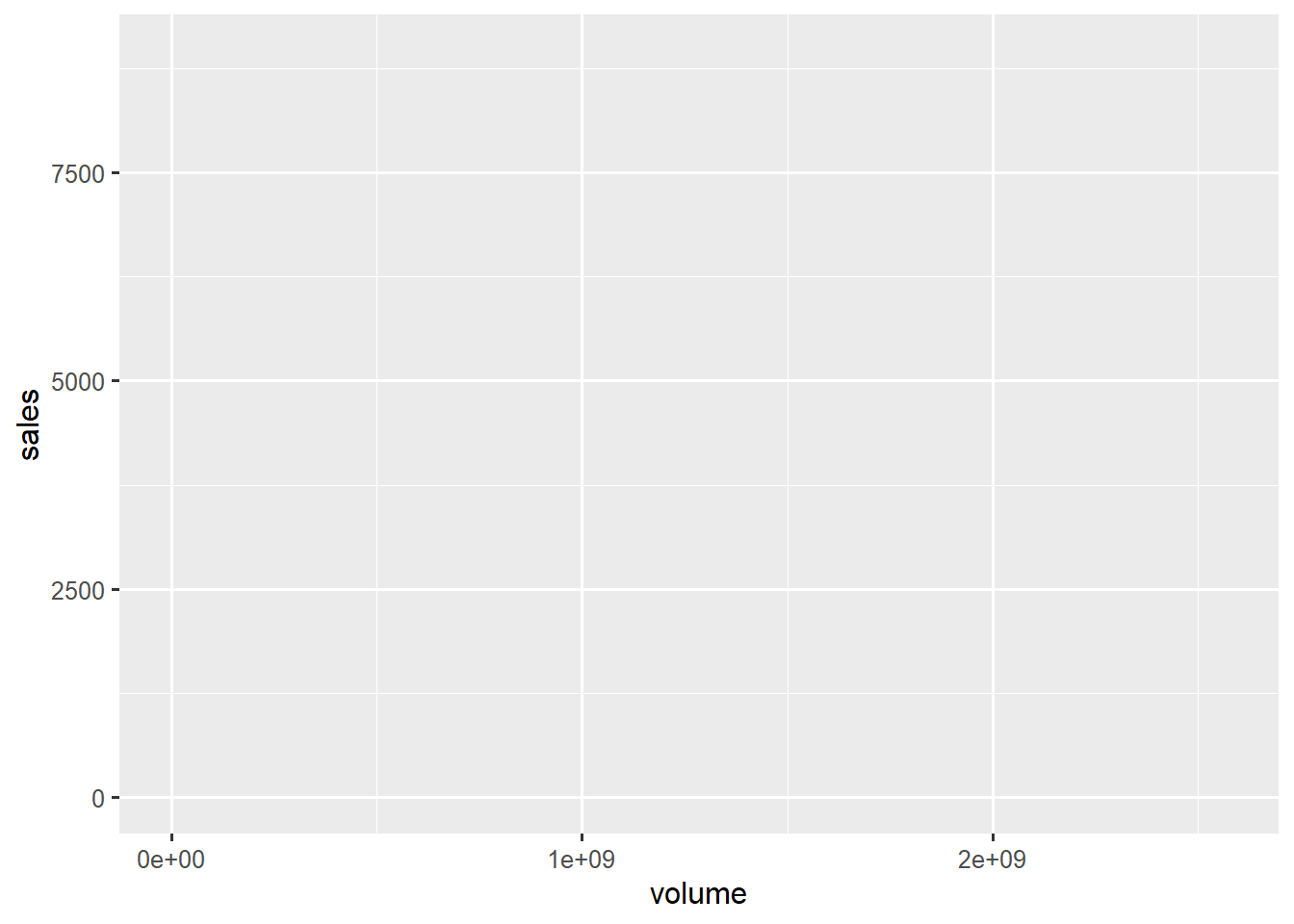without a geom or stat, just axes

We add layers with the character + to the graph to add graphical components.

Layers consist of geoms, stats, scales, and themes, which we will discuss in detail.

Remember that each subsequent layer inherits its aesthetics from ggplot(). However, specifying new aesthetics in a layer will override the aesthetics speficied in ggplot().

# scatter plot of volume vs sales
#  with rug plot colored by median sale price
ggplot(txhousing, aes(x=volume, y=sales)) +     # x=volume and y=sales inherited by all layers
geom_point() +
geom_rug(aes(color=median))   # color will only apply to the rug plot because not specified in ggplot()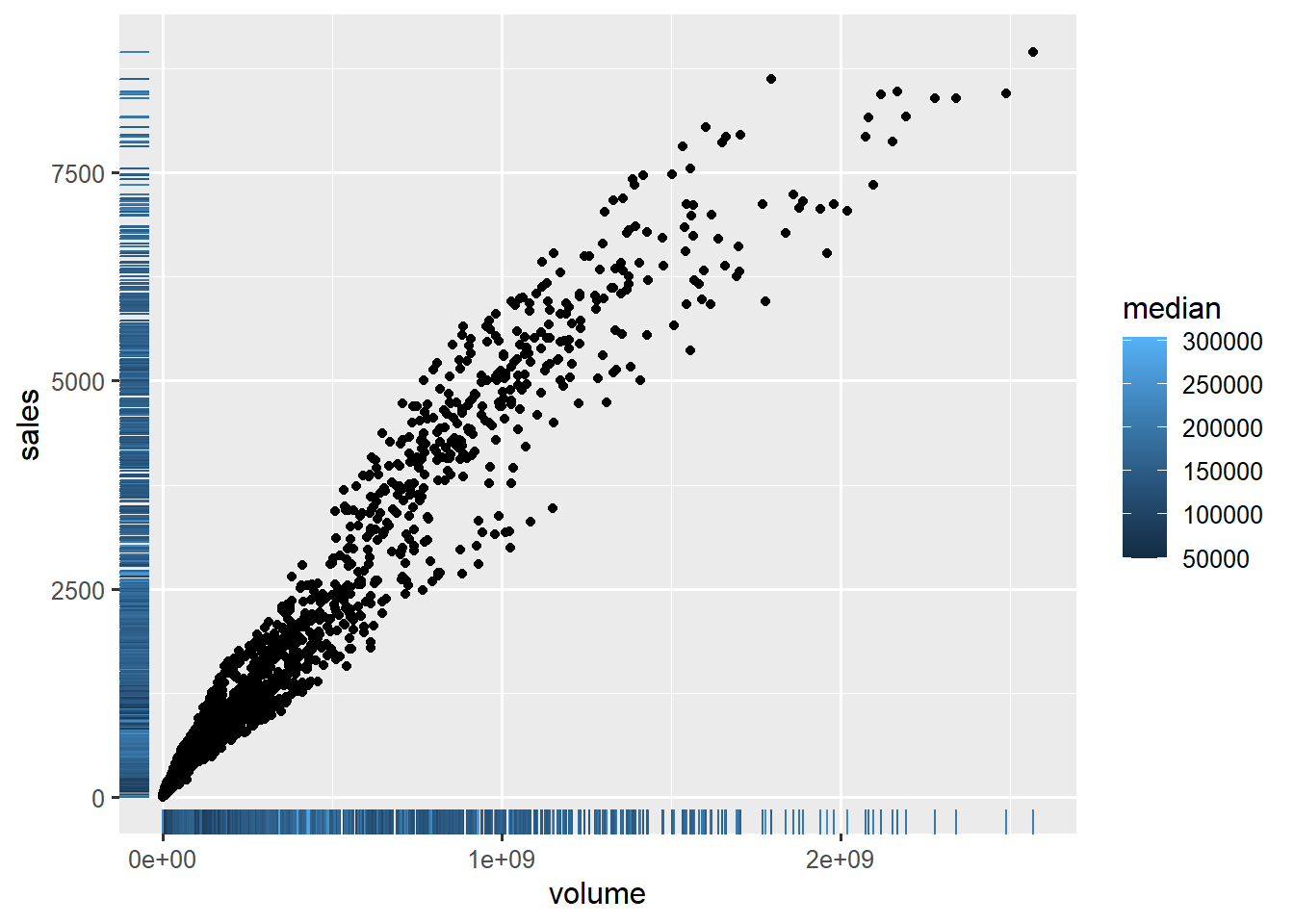both geoms inherit aesthetics from gglot, but geom_rug() also adds color aesthetic

Add a geom_point() layer to the Sitka graph we just initiated.

Add an additional geom_smooth() layer to the graph.

Both geom layers inherit x and y aesthetics from ggplot().

Specify aes(color=treat) inside of geom_point().

Notice that the coloring only applies to geom_point().

# Aesthetics

Aesthetics are the visual properties of objects on the graph.

Which aesthetics are required and which are allowed vary by geom.

Commonly used aesthetics:

• x: positioning along x-axis
• y: positioning along y-axis
• color: color of objects; for 2-d objects, the color of the object’s outline (compare to fill below)
• fill: fill color of objects
• linetype: how lines should be drawn (solid, dashed, dotted, etc.)
• shape: shape of markers in scatter plots
• size: how large objects appear
• alpha: transparency of objects (value between 0, transparent, and 1, opaque – inverse of how many stacked objects it will take to be opaque)

Change the aesthetic color mapped to treat in our previous graph to shape.

# Mapping vs setting

Map aesthetics to variables inside the aes() function. By mapping, we mean the aesthetic will vary as the variable varies. For example, mapping x=time causes the position of the plotted data to vary with values of variable “time”. Similary, mapping color=group causes the color of objects to vary with values of variable “group”.

# mapping color to median inside of aes()
ggplot(txhousing, aes(x=volume, y=sales)) +
geom_point(aes(color=median))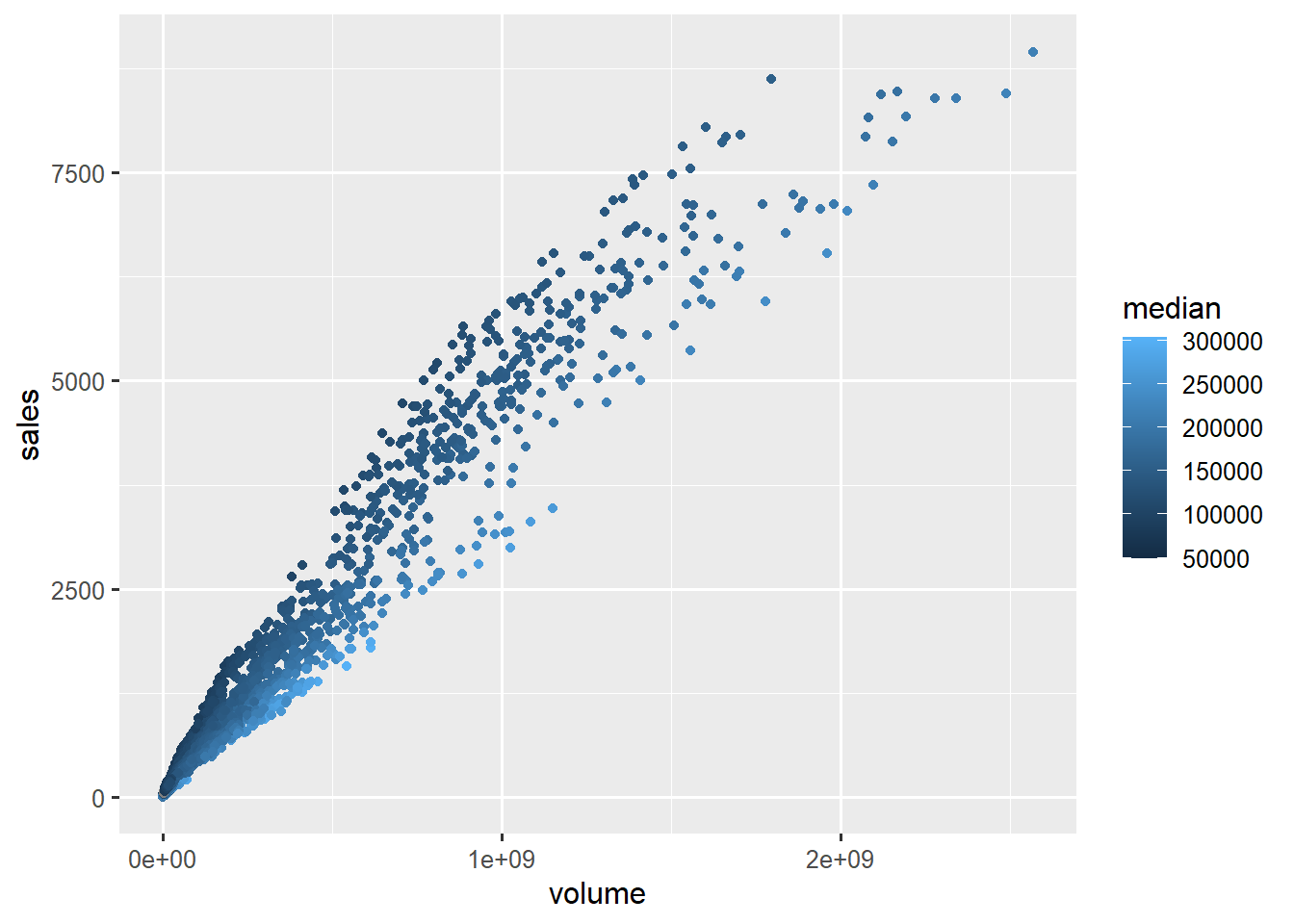color of points varies with median price

Set aesthetics to a constant outside the aes() function.

Compare the following graphs:

# setting color to green outside of aes()
ggplot(txhousing, aes(x=volume, y=sales)) +
geom_point(color="green")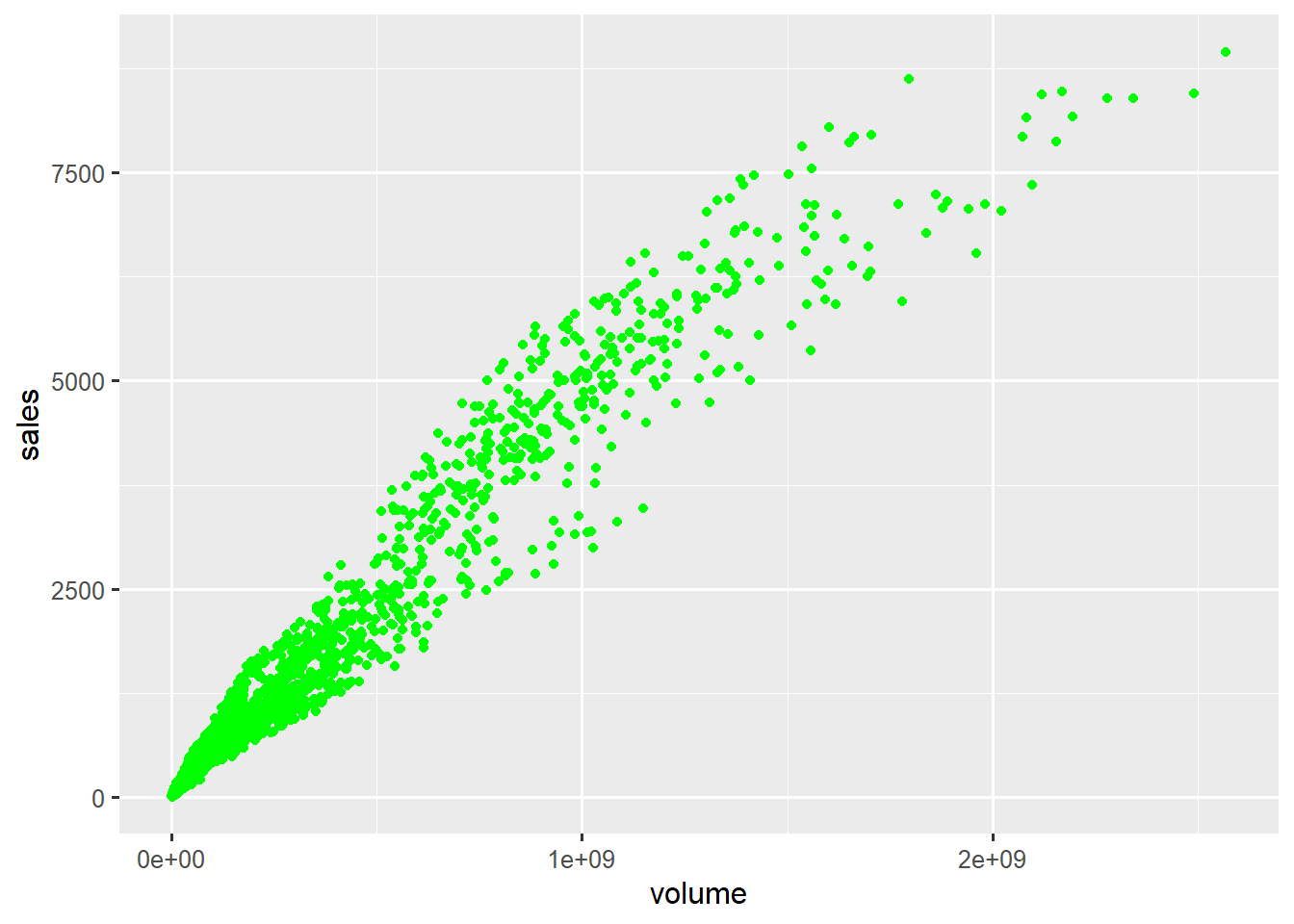color of points set to constant green

Create a new graph for data set Sitka, a scatter plot of Time (x-axis) vs size (y-axis), where all the points are colored “green”.

Setting an aesthetic to a constant within aes() can lead to unexpected results, as the aesthetic is then set to a default value rather than the specified value.

# color="green" inside of aes()
# geom_point() cannot find a variable called "green" and
#   uses a default color instead
ggplot(txhousing, aes(x=volume, y=sales)) +
geom_point(aes(color="green"))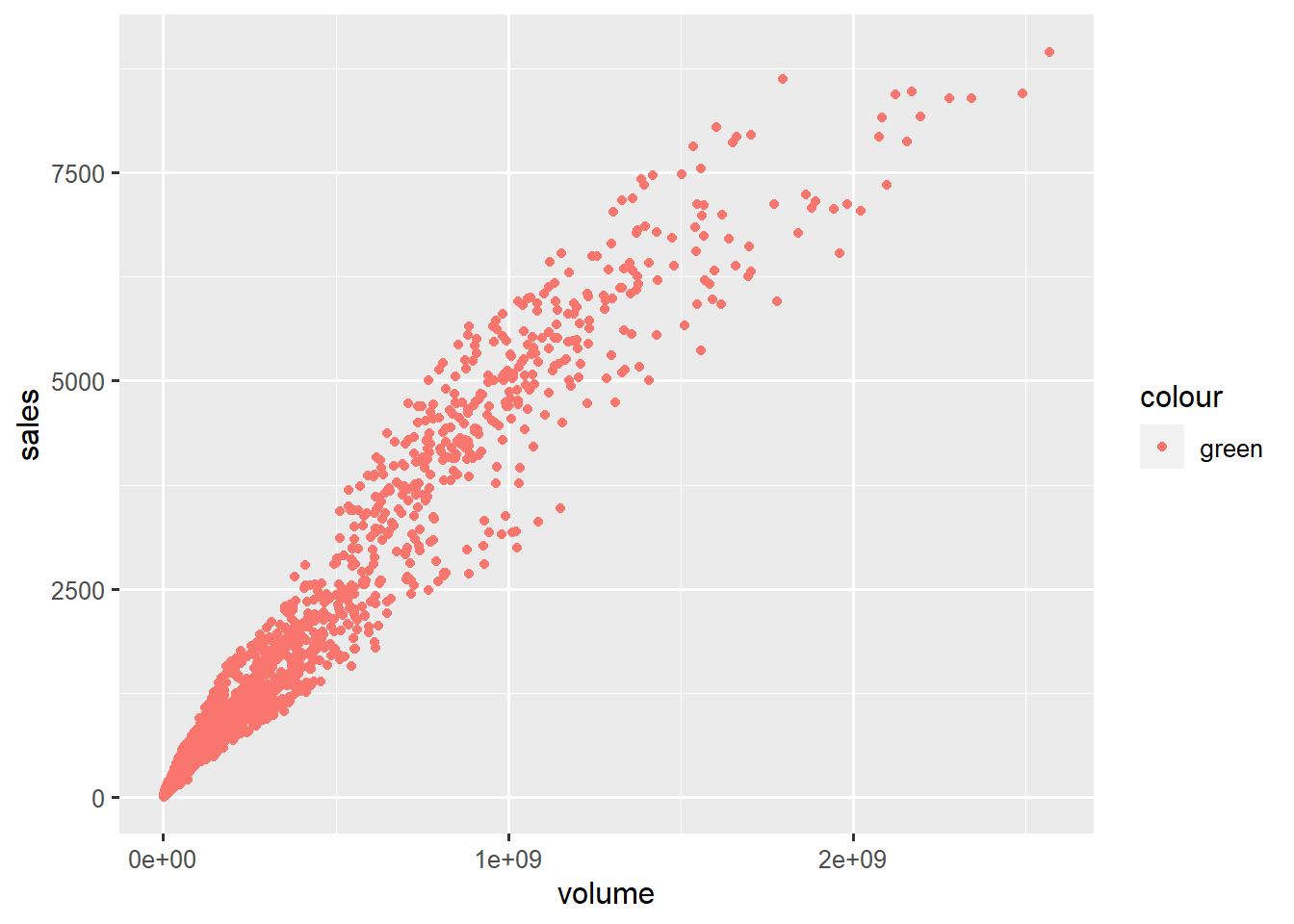aesthetic set to constant within aes() leads to unexpected results

# Geoms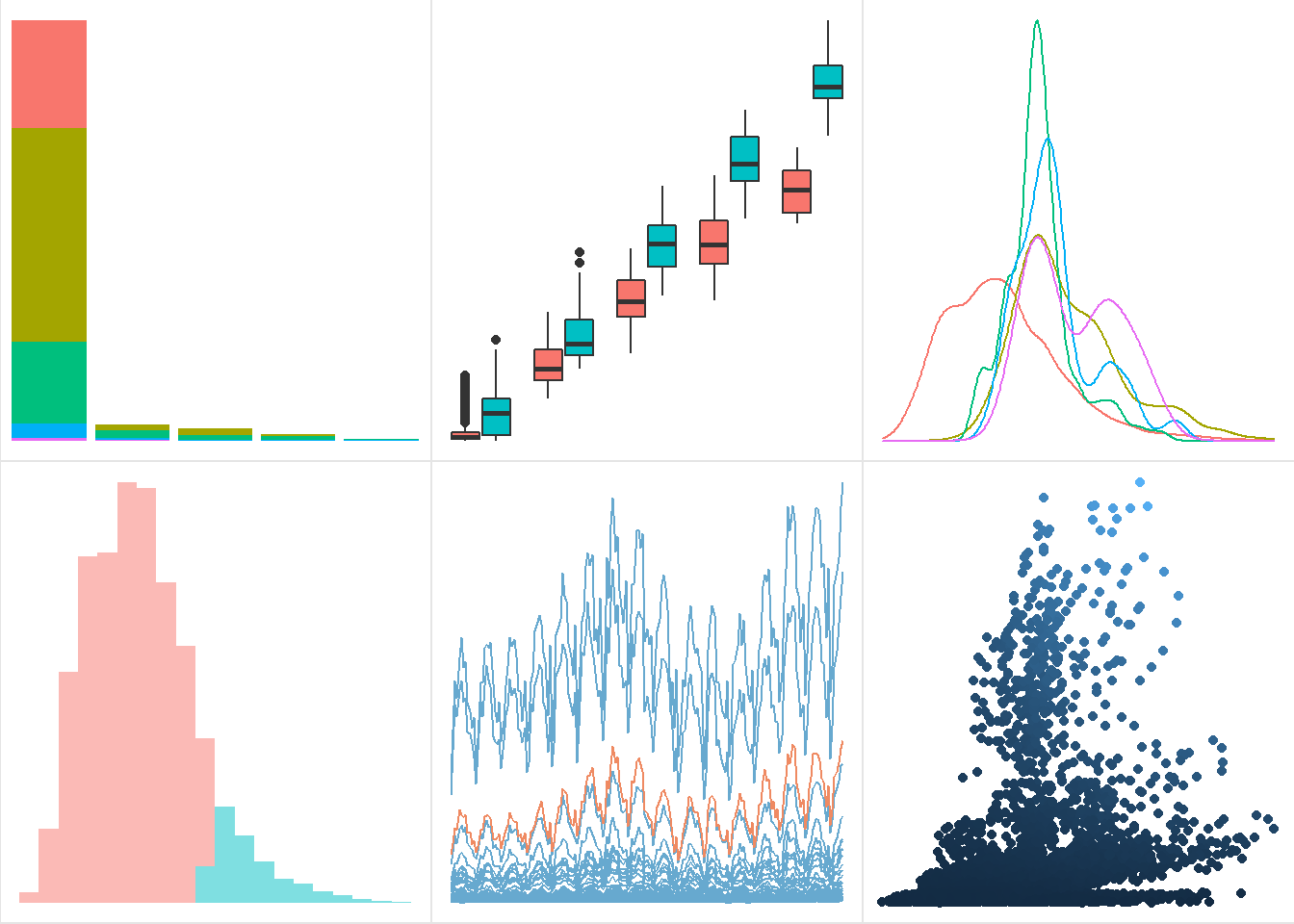geoms: bar, boxplot, density, histogram, line, point

Geom functions differ in the geometric shapes produced for the plot.

Some example geoms:

• geom_bar(): bars with bases on the x-axis
• geom_boxplot(): boxes-and-whiskers
• geom_errorbar(): T-shaped error bars
• geom_density(): density plots
• geom_histogram(): histogram
• geom_line(): lines
• geom_point(): points (scatterplot)
• geom_ribbon(): bands spanning y-values across a range of x-values
• geom_smooth(): smoothed conditional means (e.g. loess smooth)
• geom_text(): text

# Geoms and aesthetics

Each geom is defined by aesthetics required for it to be rendered. For example, geom_point() requires both x and y, the minimal specification for a scatterplot.

Geoms differ in which aesthetics they accept as arguments. For example, geom_point() accepts the aesthetic shape, which defines the shapes of points on the graph, while geom_bar() does not accept shape.

Check the geom function help files for required and understood aesthetics. In the Aesthetics section of the geom’s help file, required aesthetics are bolded.

We will tour some commonly used geoms.

# Histograms

ggplot(txhousing, aes(x=median)) +
geom_histogram()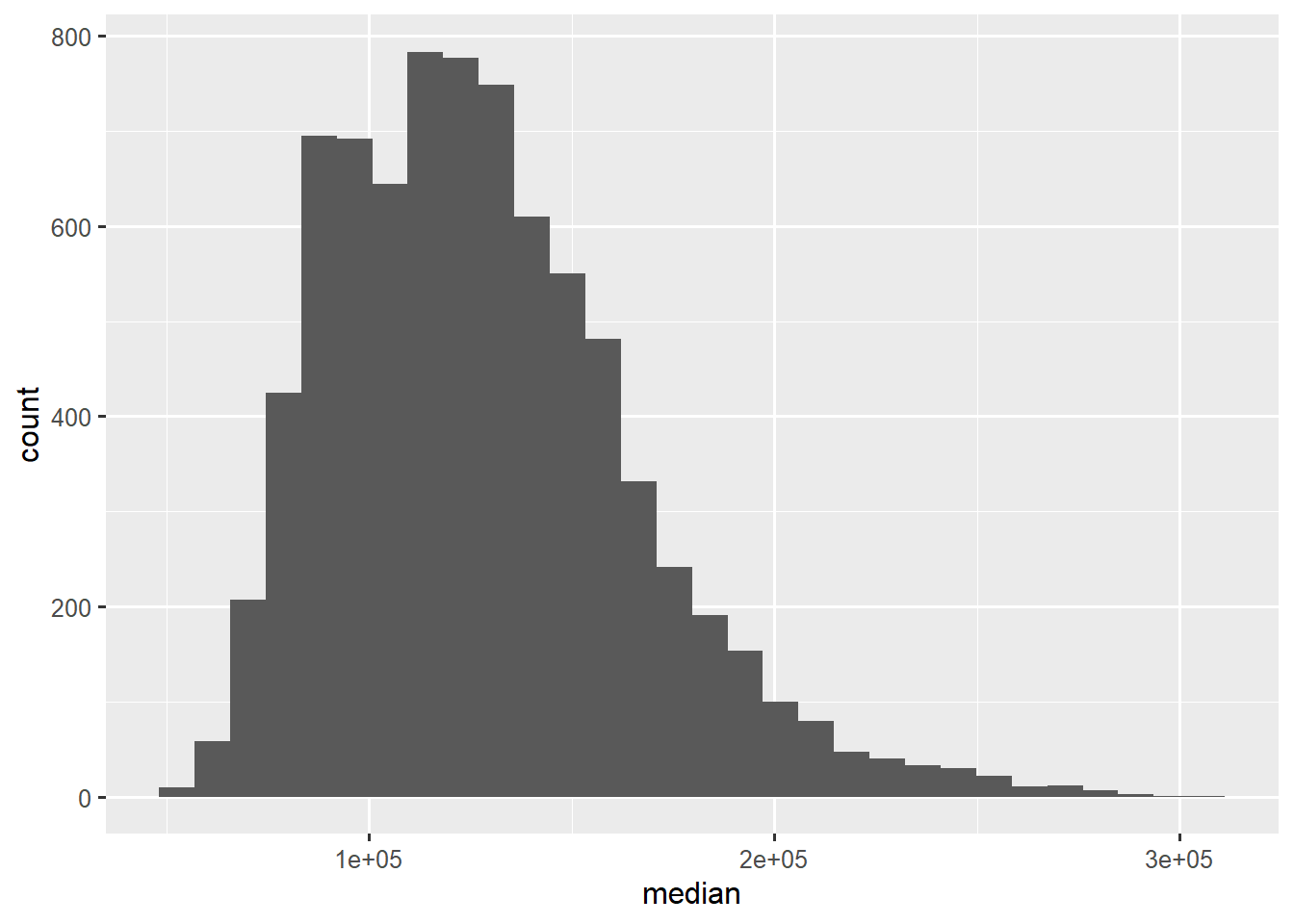histograms visualize distribution of variable mapped to x

Histograms are popular choices to depict the distribution of a continuous variable.

geom_histogram() cuts the continuous variable mapped to x into bins, and count the number of values within each bin.

Create a histogram of size from data set Sitka.

ggplot2 issues a message urging you to pick a number of bins for the histogram (it defaults to 30), using the bins argument.

Specify bins=20 inside of geom_histogram(). Note: bins is not an aesthetic, so should not be specified within aes().

# Density plots

ggplot(txhousing, aes(x=median)) +
geom_density()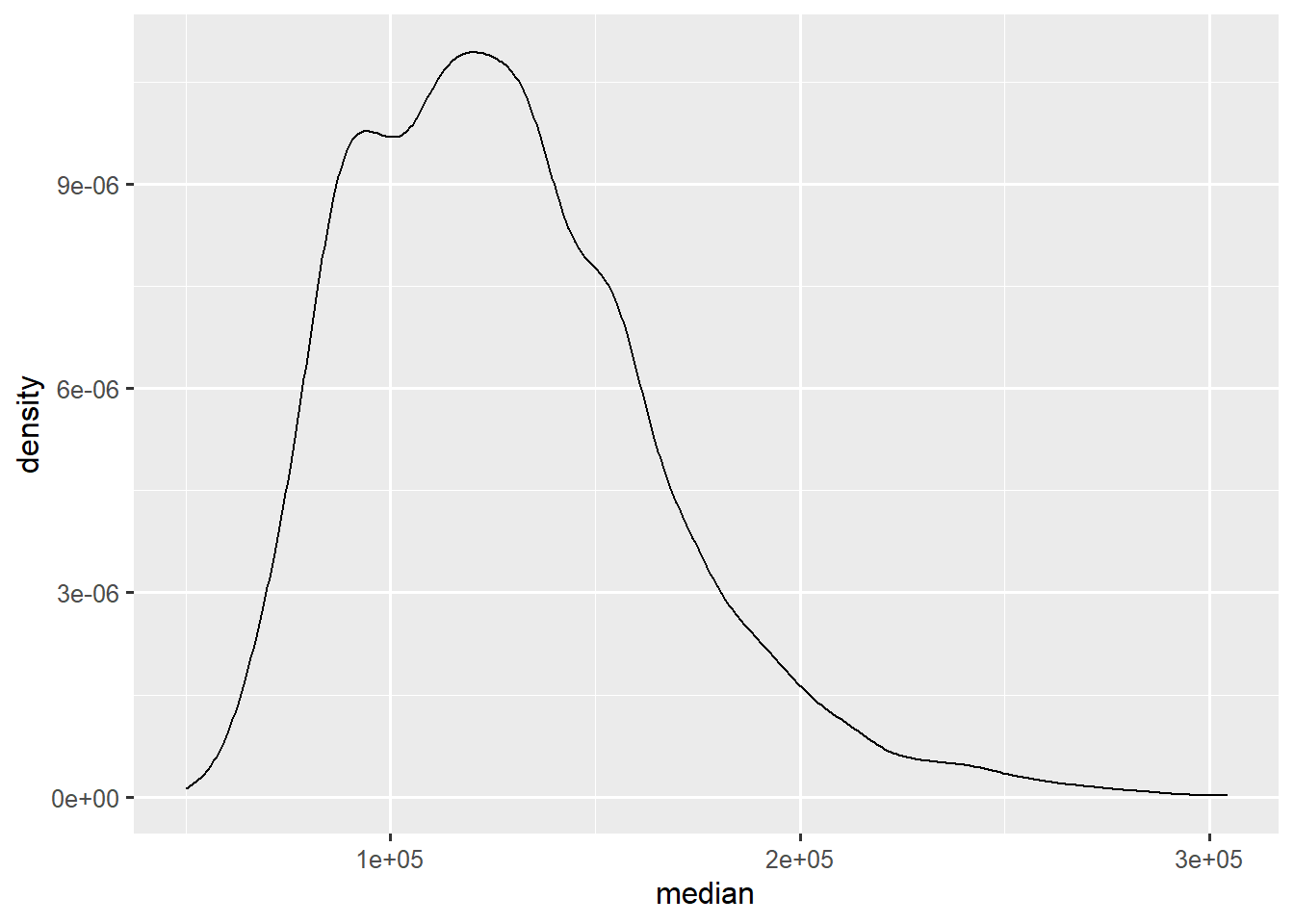density plots visualize smoothed distribution of variable mapped to x

Denisty plots are basically smoothed histograms.

Density plots, unlike histograms, can be plotted separately by group by mapping a grouping variable to color.

ggplot(txhousing, aes(x=median, color=factor(month))) +
geom_density()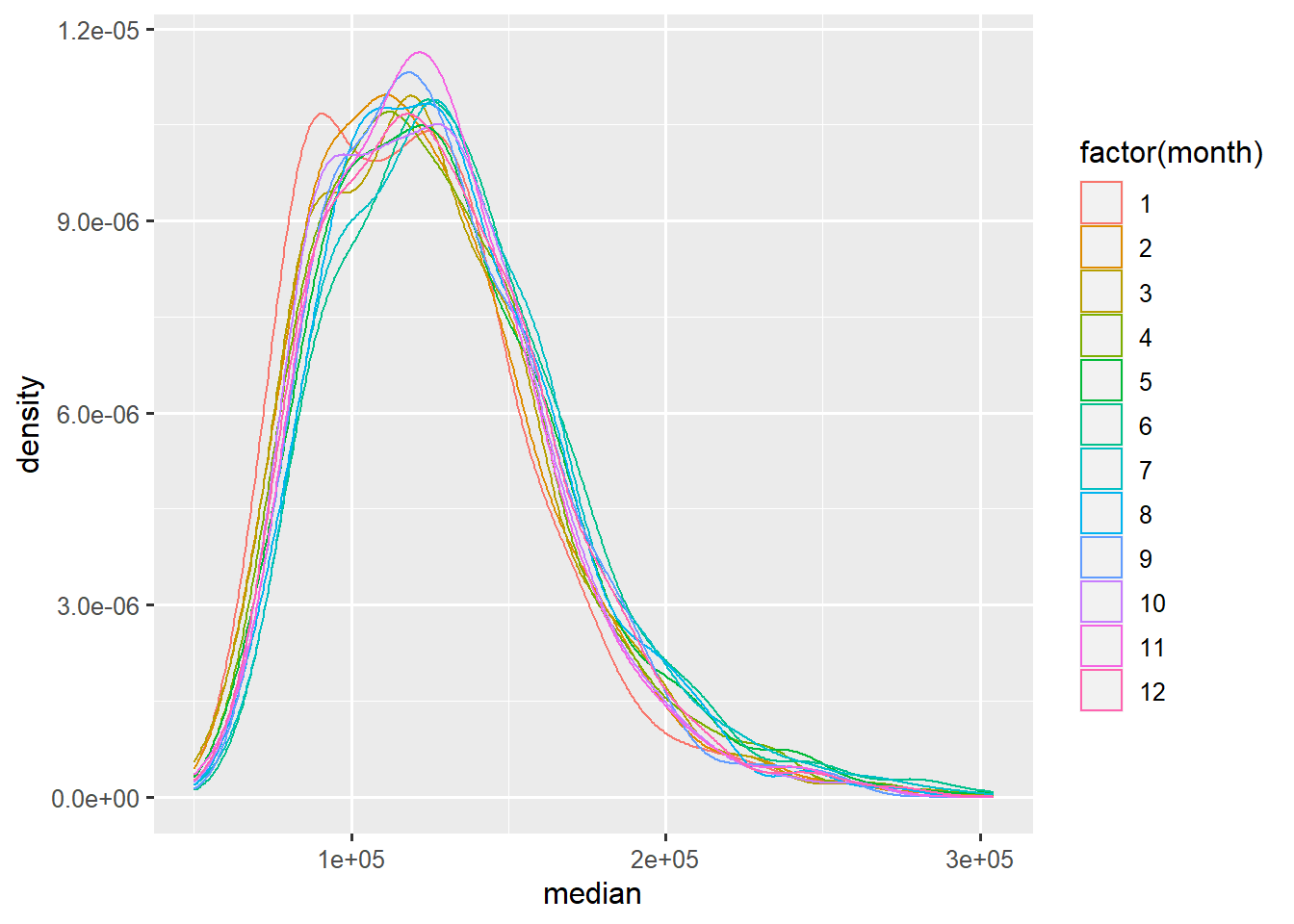densities of median price by month

# Boxplots

ggplot(txhousing, aes(x=factor(year), y=median)) +
geom_boxplot()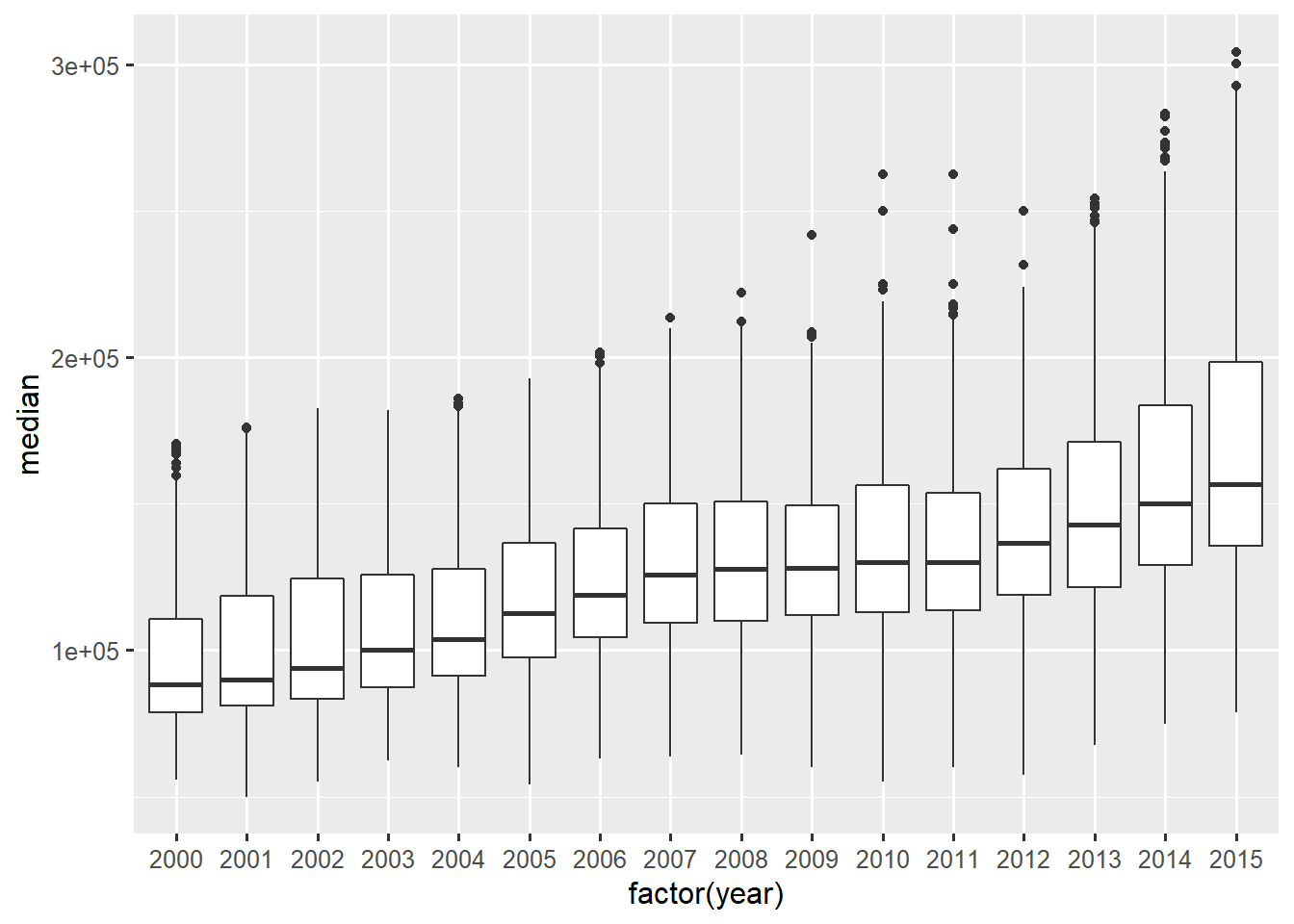boxplots are useful to compare distribution of y variable across levels of x variable

Boxplots compactly visualize particular statistics of a distributions:

• lower and upper hinges of box: first and third quartiles
• middle line: median
• lower and upper whiskers: $$(hinge - 1.5 \times IQR)$$ and $$(hinge + 1.5 \times IQR)$$ where $$IQR$$ is the interquartile range (distance between hinges)
• dots: outliers

Boxplots are perhaps are particularly useful for comparing whole distributions of a continuous variable between groups.

geom_boxplot() will create boxplots of the variable mapped to y for each group defined by the values of the x variable.

Create a new graph where we compare distributions of size across levels of treat from dataset Sitka.

# Bar plots

ggplot(diamonds, aes(x=cut)) +
geom_bar()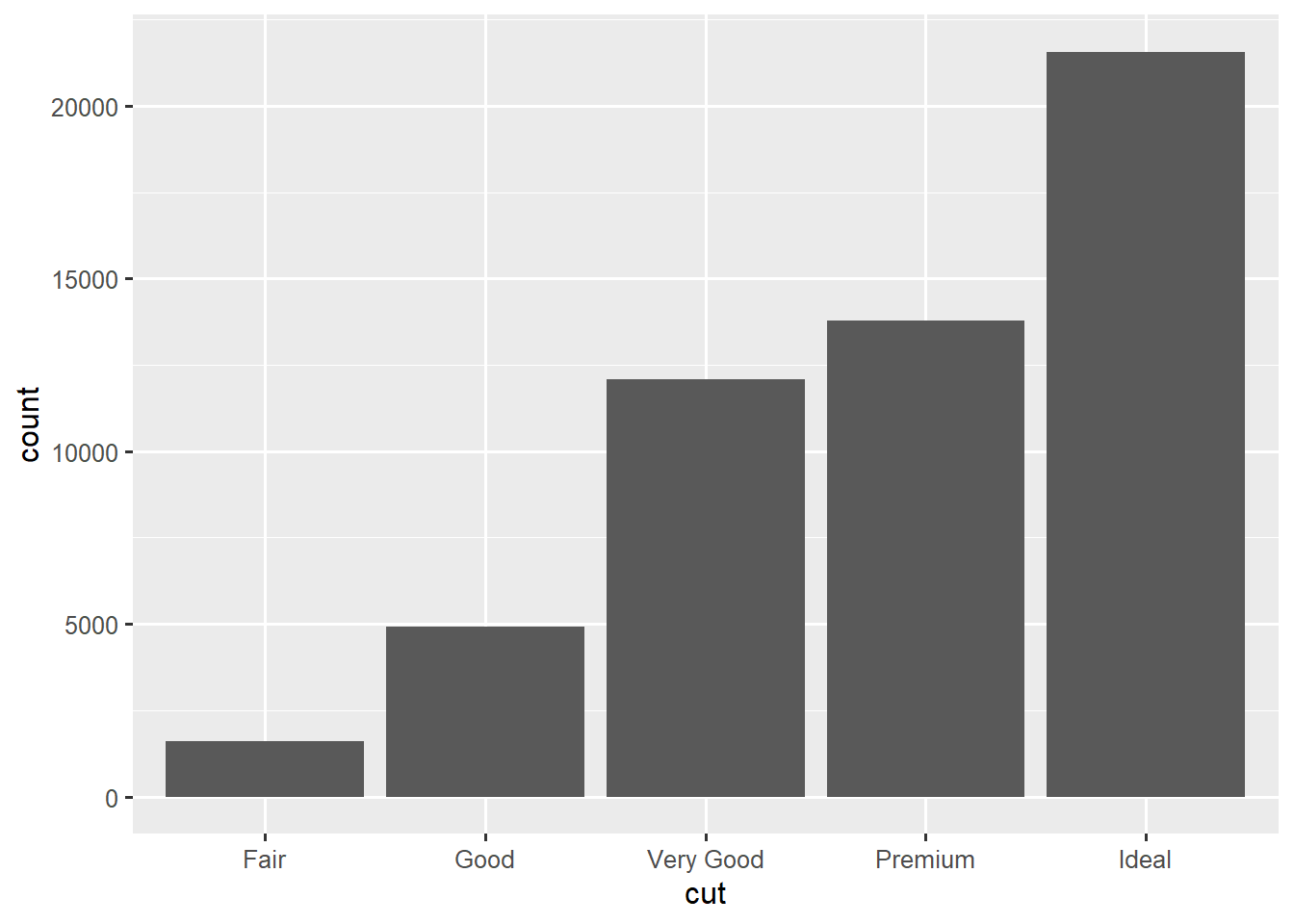geom_bar displays frequencies of levels of x variable

Bar plots are often used to display frequencies of factor (categorical) variables.

geom_bar() by default produces a bar plot where the height of the bar represents counts of each x-value.

Start a new graph where the frequencies of treat from data set Sitka are displayed as a bar graph. Remember to map x to treat.

The color that fills the bars is not controlled by aesthetic color, but instead by fill, which can only be mapped to a factor (categorical) variable. We can visualize a crosstabulation of variables by mapping one of them to fill in geom_bar():

ggplot(diamonds, aes(x=cut, fill=clarity)) +
geom_bar()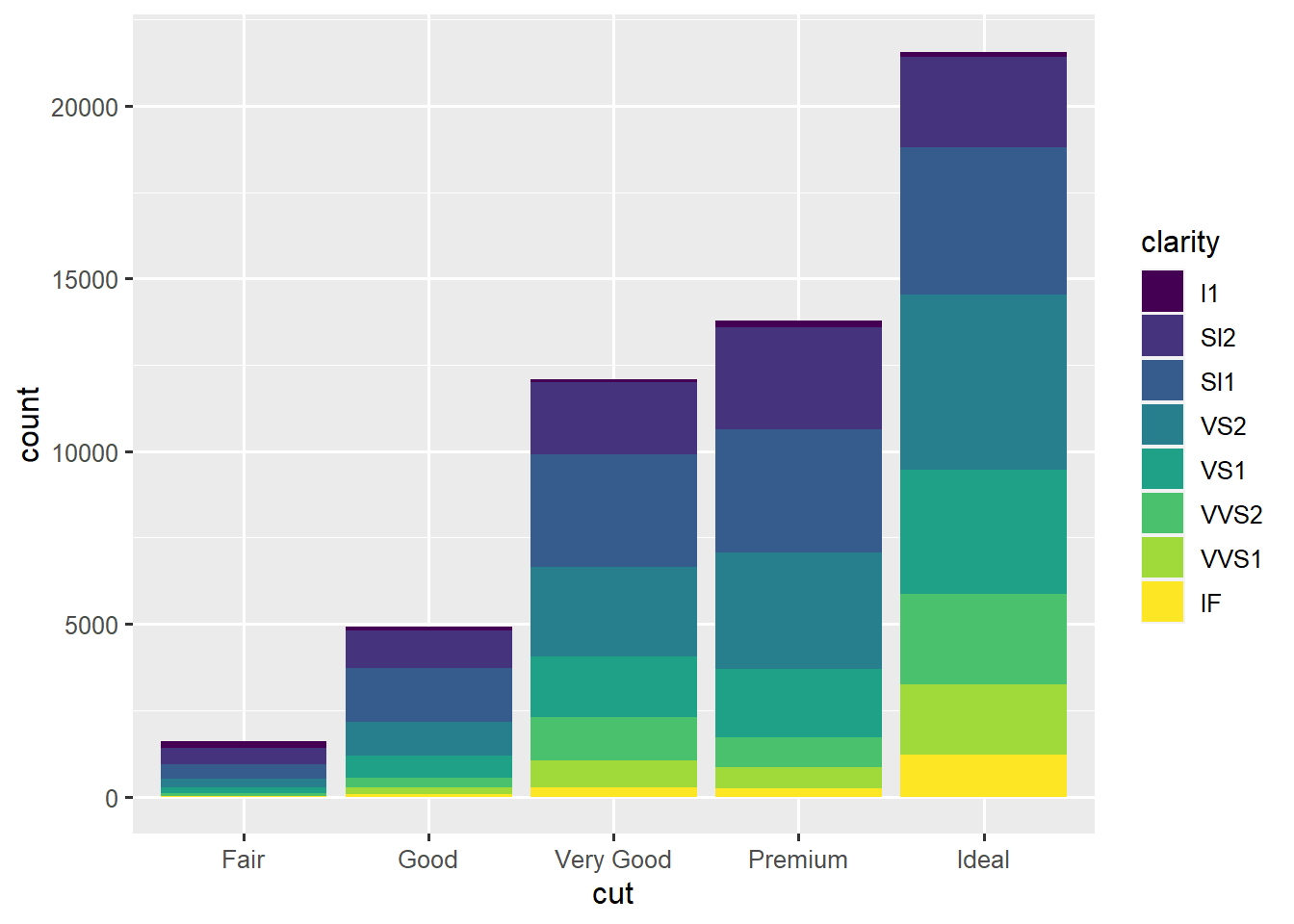frequencies of cut by clarity

Add the aesthetic mapping fill=factor(Time) to aes() inside of ggplot() of the previous graph.

# Scatter plots

# scatter of volume vs sales
ggplot(txhousing, aes(x=volume, y=sales)) +
geom_point()scatter plot of volume vs sales

Scatter plots depict the covariation between pairs of variables (typically both continuous).

geom_point() depicts covariation between variables mapped to x and y.

Scatter plots are among the most flexible graphs, as variables can be mapped to many aesthetics such as color, shape, size, and alpha.

ggplot(txhousing, aes(x=volume, y=sales,
color=median, alpha=listings, size=inventory)) +
geom_point()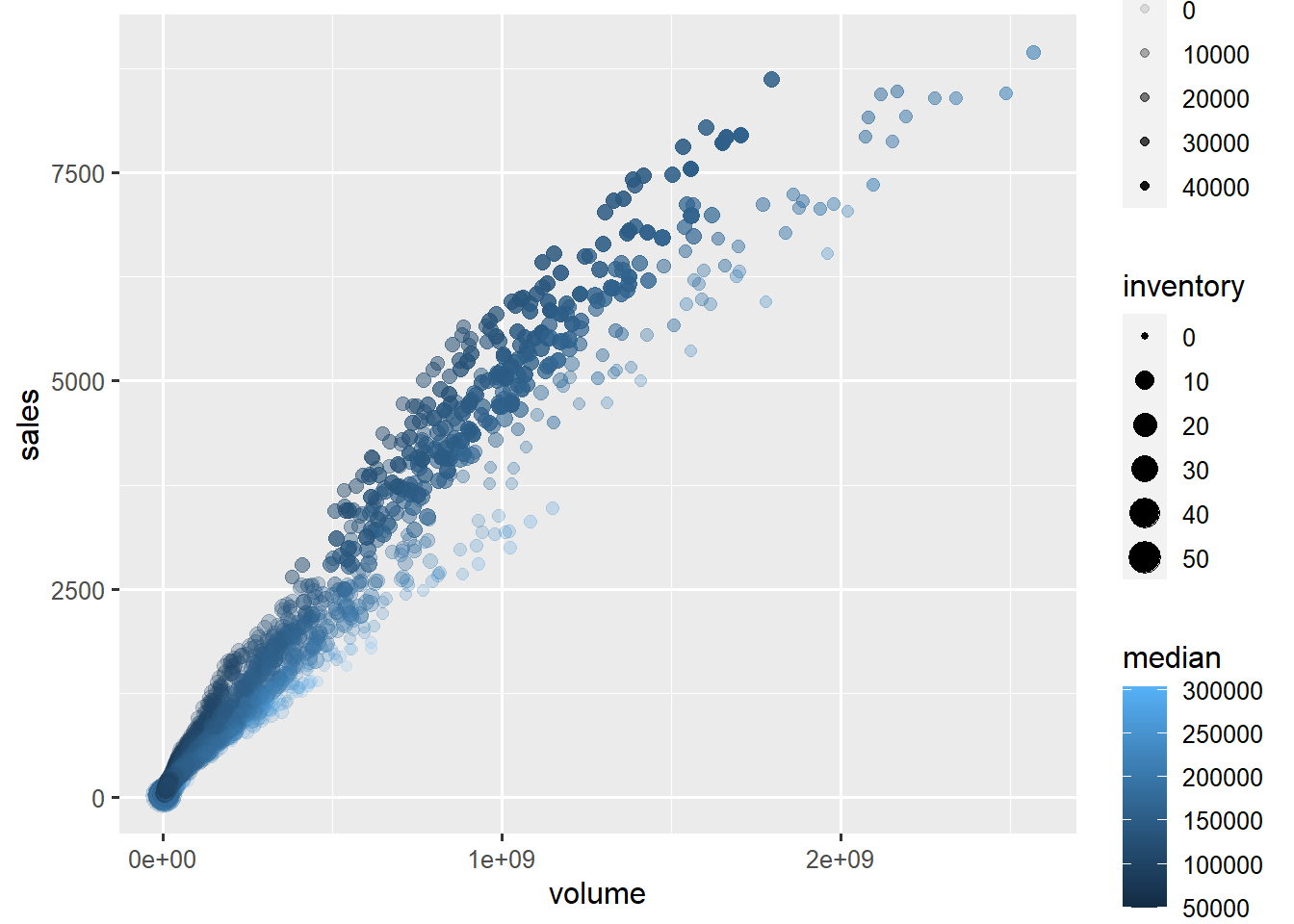scatter plot of volume vs sales, colored by median price, transparent by number of listings, and sized by inventory

# Line graphs

ggplot(txhousing, aes(x=date, y=sales, group=city)) +
geom_line()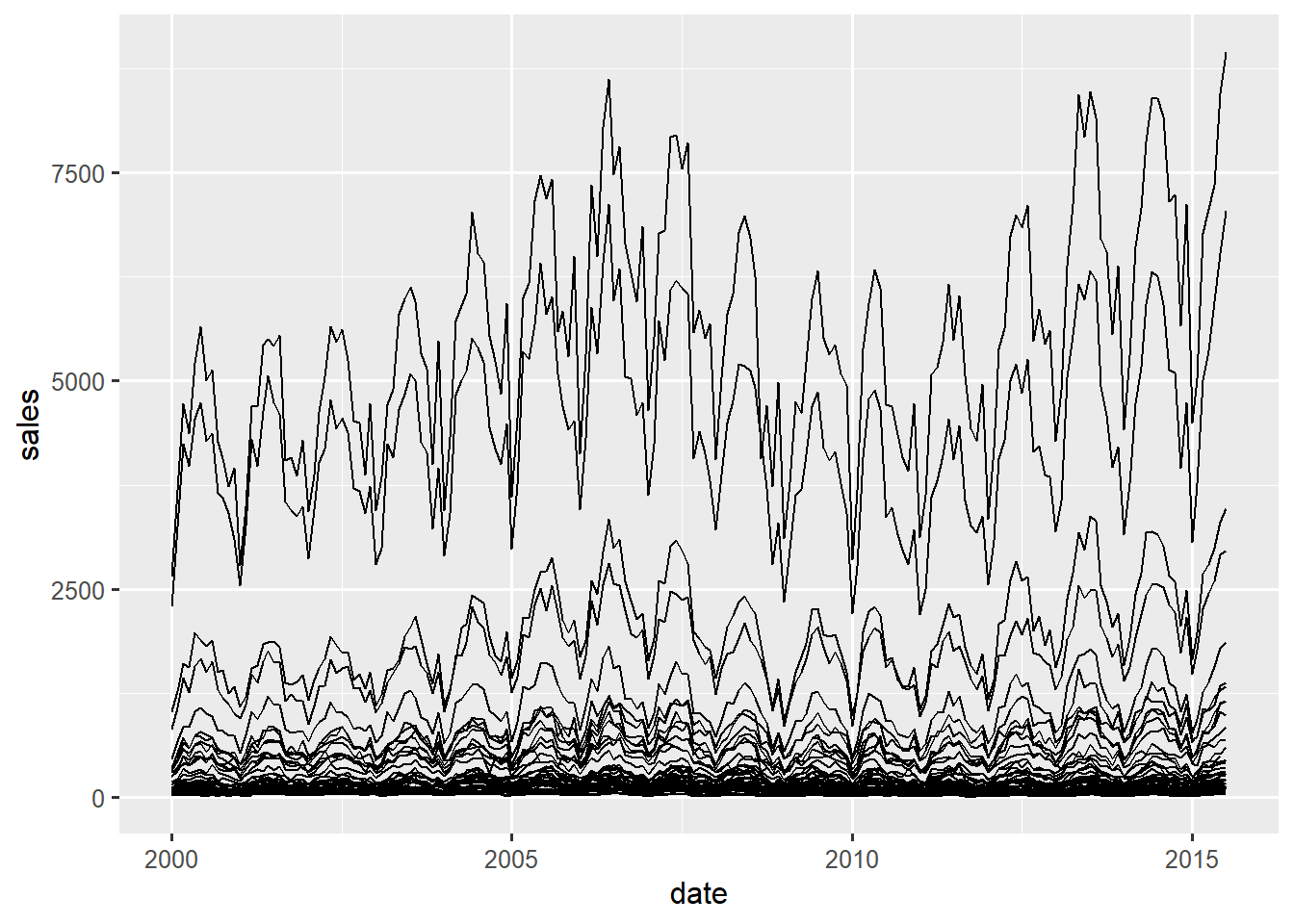line graph of sales over time, separate lines by city

Line graphs depict covariation between variables mapped to x and y with lines instead of points.

geom_line() will treat all data as belonging to one line unless a variable is mapped to one of the following aesthetics to group the data into separate lines:

• group: lines will look the same
• color: line colors will vary with mapped variable
• linetype: line patterns will vary with mapped variable

Let’s first examine a line graph with no grouping:

ggplot(txhousing, aes(x=date, y=sales)) +
geom_line()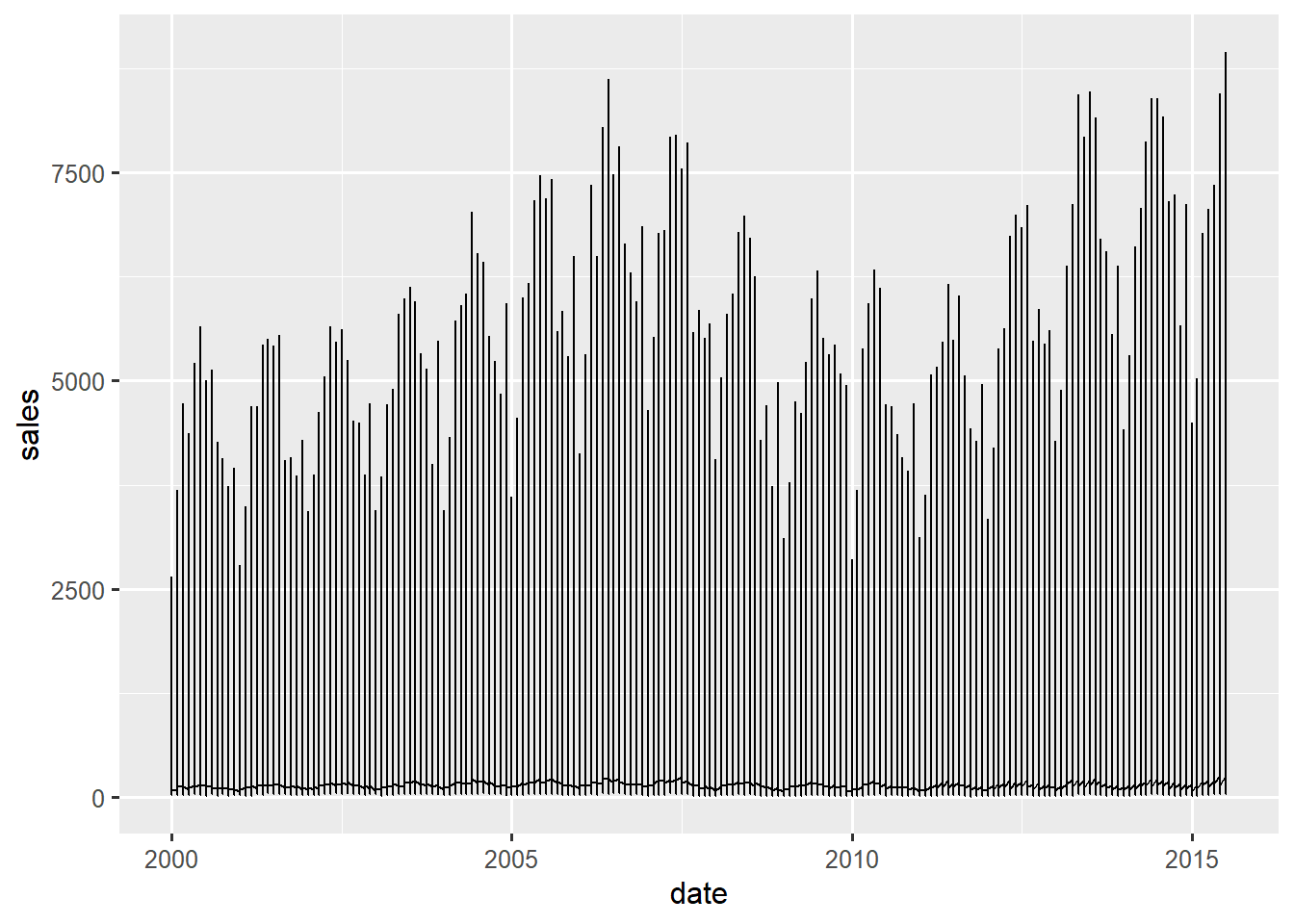line graph of sales over time, no grouping results in garbled graph

As you can see, unless the data represent a single series, line graphs usually call for some grouping.

Using color or linetype in geom_line() will implicitly group the lines.

ggplot(txhousing, aes(x=date, y=sales, color=city)) +
geom_line()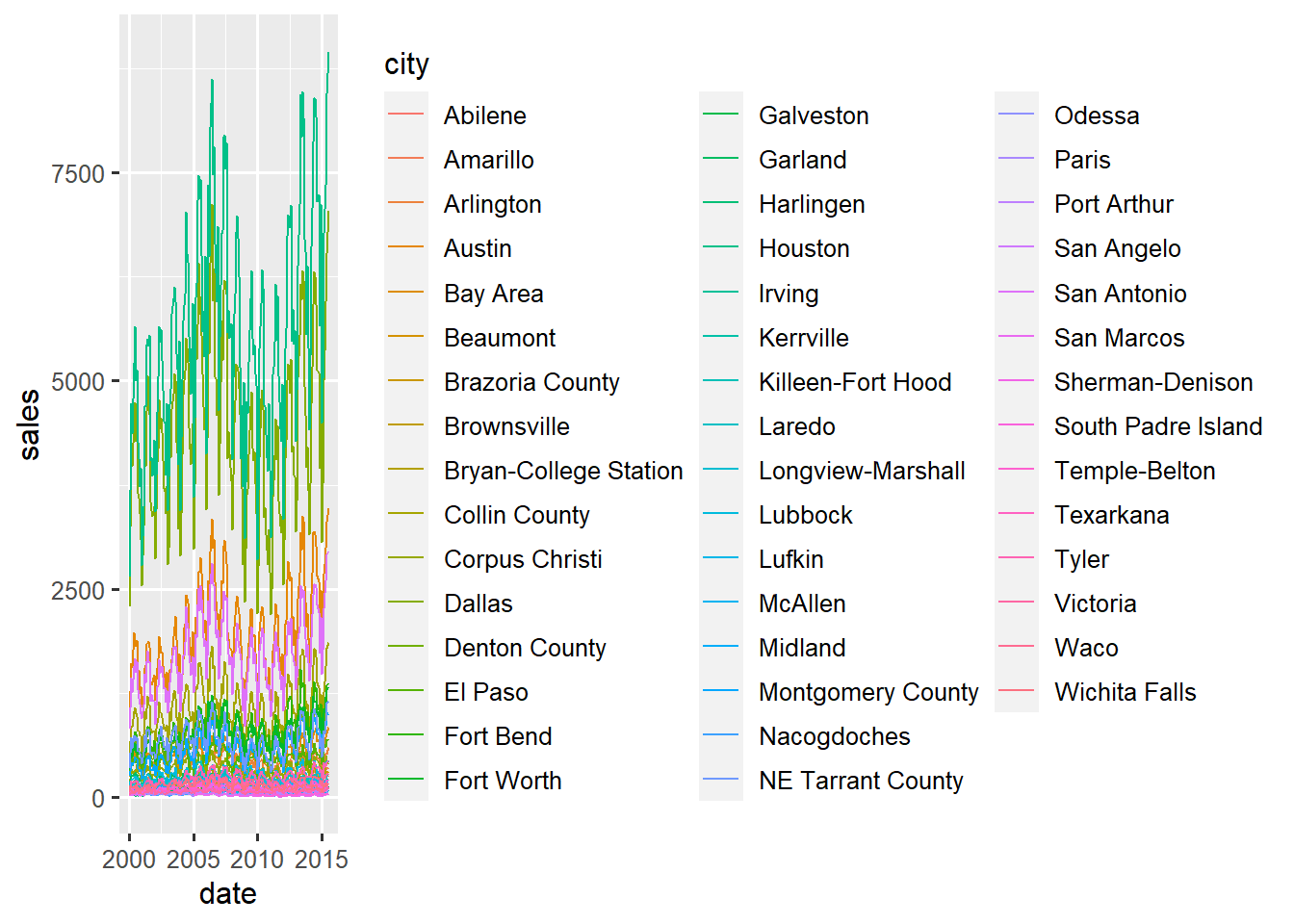line graph of sales over time, colored and grouped by city

Let’s try graphing separate lines (growth curves) for each tree.

Create a new line graph for data set Sitka with Time on the x-axis and size on the y axis, but also map group to tree.

We can specify color and linetype in addition to group. The lines will still be separately drawn by group, but can be colored or patterned by additional variables.

In our data, We might want to compare trajectories of growth between treatments.

Now add a specification mapping color to treat (in addition to group=tree).

Finally, map treat to linetype instead of color.

# *Stats*

The stat functions statistically transform data, usually as some form of summary, such as the mean, or standard devation, or a confidence interval.

Each stat function is associated with a default geom, so no geom is required for shapes to be rendered.

stat_summary(), perhaps the most useful of all stat functions, applies a summary function to the variable mapped to y for each value of the x variable. The default summary function is mean_se(), with associated geom geom_pointrange(), which will produce a plot of the mean (dot) and standard error (lines) of the variable mapped to y for each value of the x variable.

# summarize sales (y) for each year (x)
ggplot(txhousing, aes(x=year, y=sales)) +
stat_summary()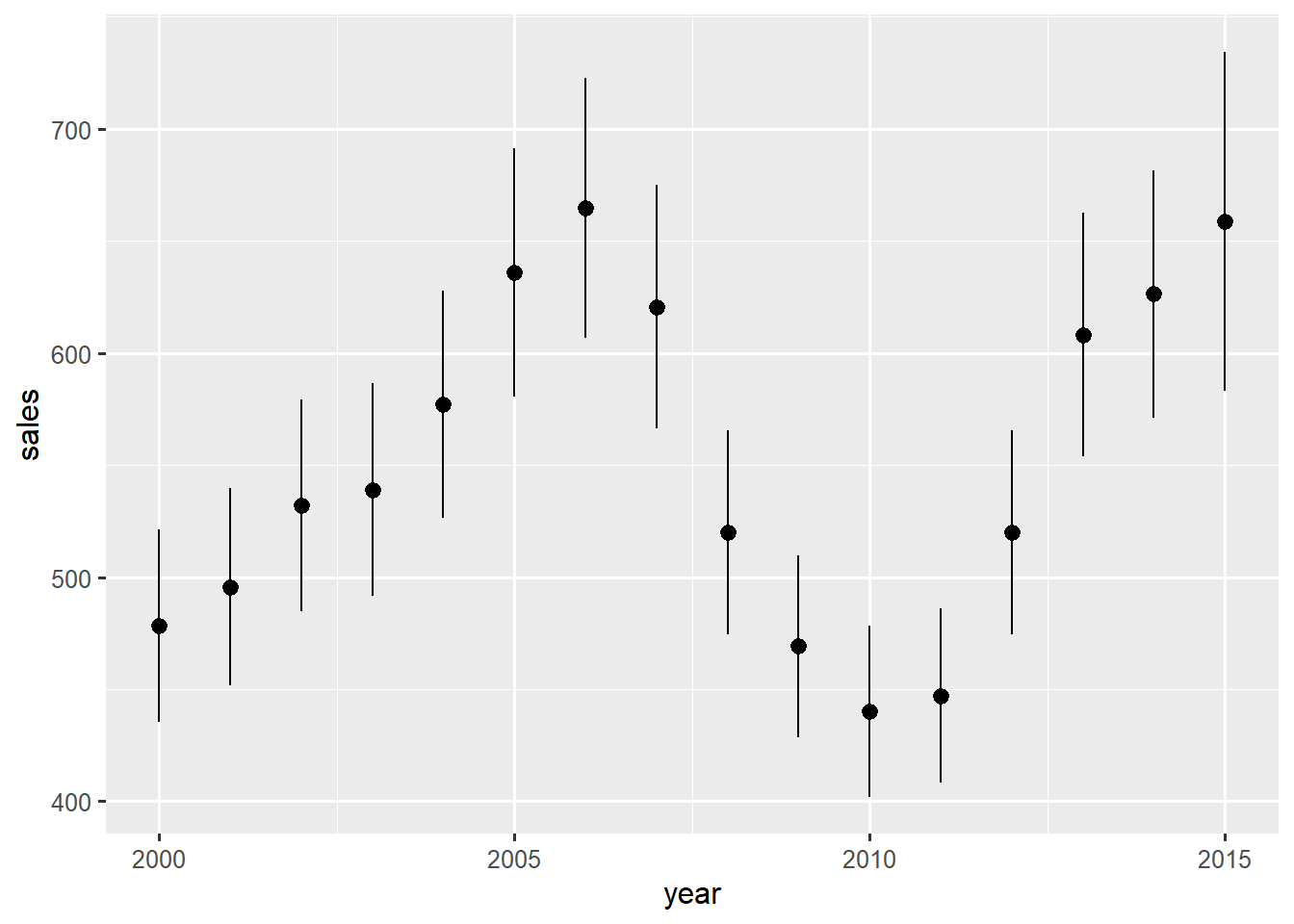mean and standard errors of sales by year

Create a new plot where x is mapped to Time and y is mapped to size. Then, add a stat_summary() layer.

What makes stat_summary() so powerful is that you can use any function that accepts a vector as the summary function (e.g. mean(), var(), max(), etc.) and the geom can also be changed to adjust the shapes plotted.

# Scales

Scales define which aesthetic values are mapped to the data values.

Here is an example of a color scale that defines which colors are mapped to values of treat:

color treat
red ozone
blue control

Imagine that we might want to change the colors to “green” and “orange”.

The scale_ functions allow the user to control the scales for each aesthetic. 0 These scale functions have names with structure scale_aesthetic_suffix, where aesthetic is the name of an aesthetic like color or shape or x, and suffix is some descriptive word that defines the functionality of the scale.

Then, to specify the aesthetic values to be used by the scale, supply a vector of values to the values argument (usually) of the scale function.

Some example scales functions:

• scale_color_manual(): define an arbitrary color scale by specifying each color manually
• scale_color_hue(): define an evenly-spaced color scale by specifying a range of hues and the number of colors on the scale
• scale_shape_manual(): define an arbitrary shape scale by specifying each shape manually

See the ggplot2 documentation page section on scales to see a full list of scale functions.

Here is a color scale that ggplot2 chooses for us:

ggplot(diamonds, aes(x=carat, y=price, color=cut)) +
geom_point()